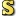﻿Multiply Method
Cove - A Practical Quantum Computer Programming Framework
Multiply Method
NamespacesCove.ClassicalUtilitiesComplexMultiply()()()Members
IconMemberDescriptionMultiply(Complex, Complex)
Perform multiplication between complex numbers. Multiplication is defined as (a + bi)(c + di) = (ac - bd) + (ad + bc)iMultiply(Double, Complex)
Perform multiplication by a number. Multiplication is defined as x(a + bi) = ax + bxiMultiply(Complex, Double)
Perform multiplication by a number. Multiplication is defined as x(a + bi) = ax + bxi

Assembly: Cove.ClassicalUtilities (Module: Cove.ClassicalUtilities) Version: 1.0.0.0 (1.0.0.0)